# laser cutting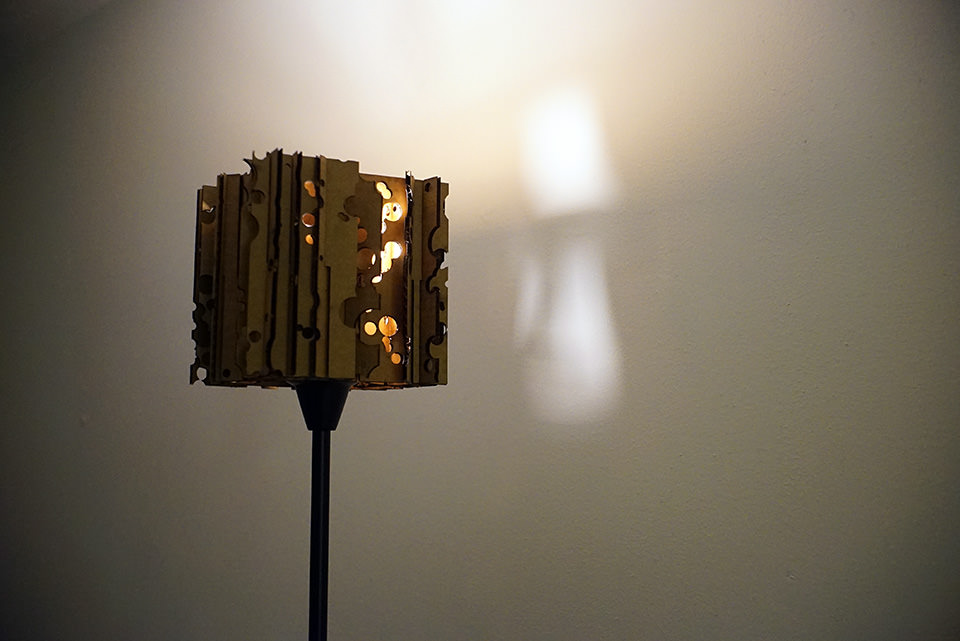I ended this week's assignment with this swiss cheese lamp shade.

The second week assignment was to make a press-fit construction kit. The first test I did was to get a clue of the width of the laser.

After experimenting and fine tunning the width, I have came up to the following settings using EPILOG at CBA shop.

• Power : 80% (~83% if you are cutting in large area)
• Speed : 20%
• Strip width : 0.149 in

I wanted to experiment different angles jointing. I thought it interesting that the joints will not be fixed independently. (less contact to gain friction)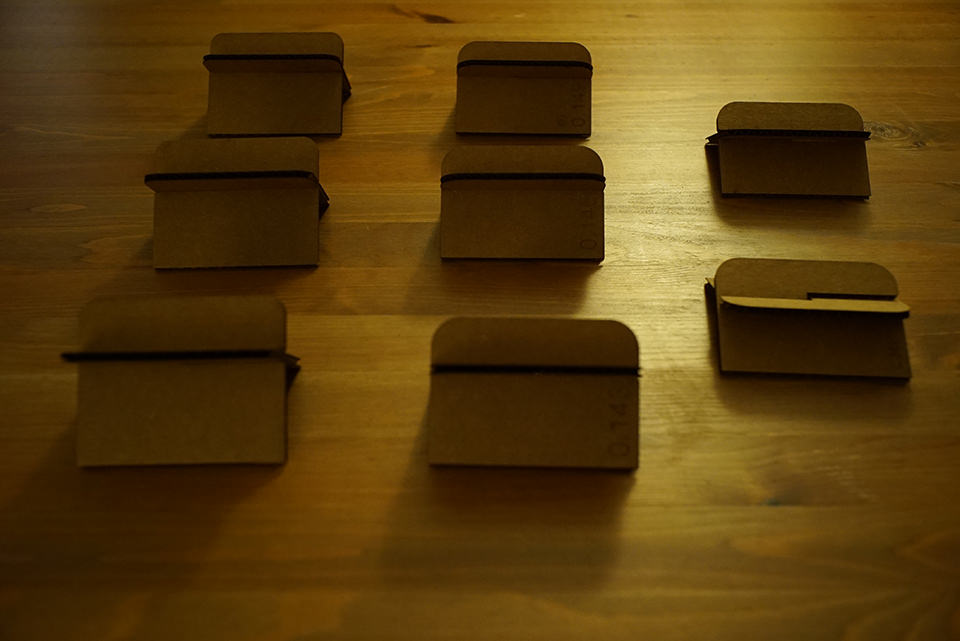Fig1. Sample cuts, different width of cutting.Fig2. The settings for a perfect fit. (0.149in using EPILOG)

Rhino+Grasshopper was used through modeling, but the majority of the process was to code and generate the shape automatically. (Fig.3 The big one in the middle is the python scripting coponent. see Appendix for a portion of the code.)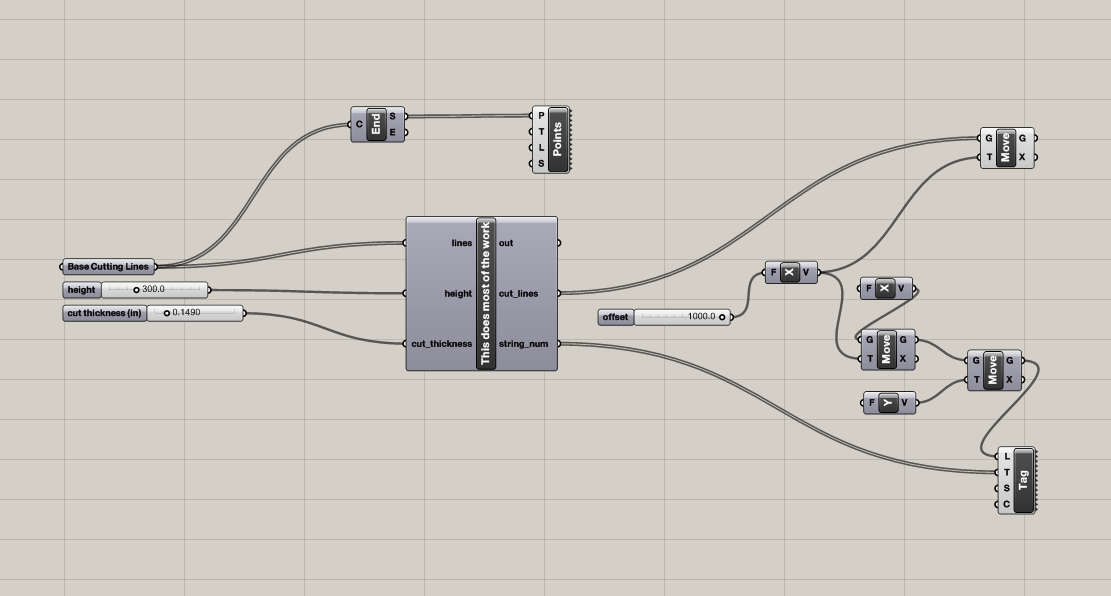Fig3. Grasshopper definition. The python component in the middle does the most of the work.

I wasn't sure if the peices will hold theirselves together, but as I was assembling, I understood that the worries were groundless.

One thing I came out good was the order of the parts. The order of the assembly was critical, because the nature of interlocking. The script did that for me, but I wasn't aware of that when I was using CAD.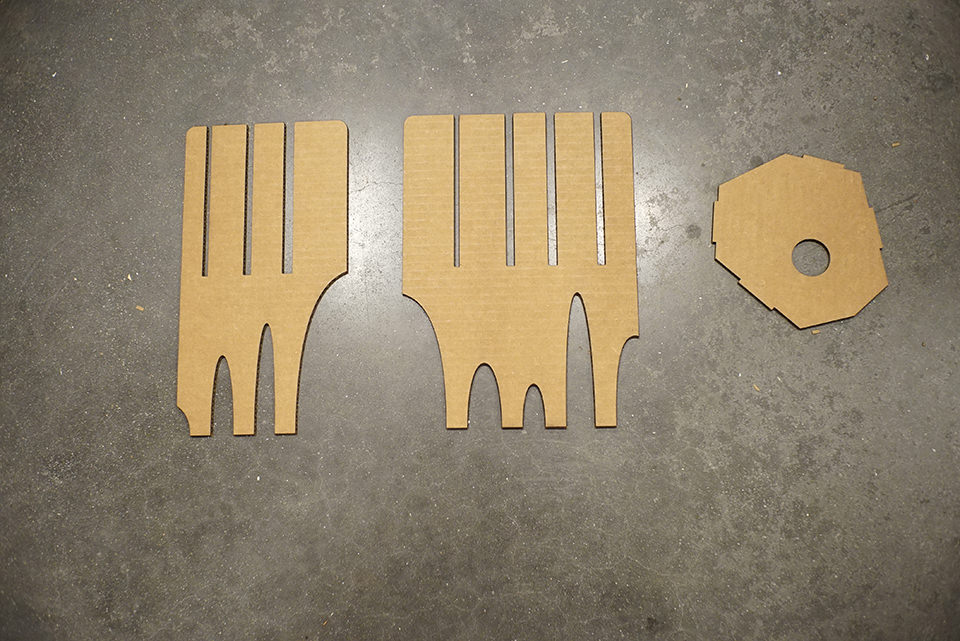Fig4. Sample of parts. Right peice for fitting the light.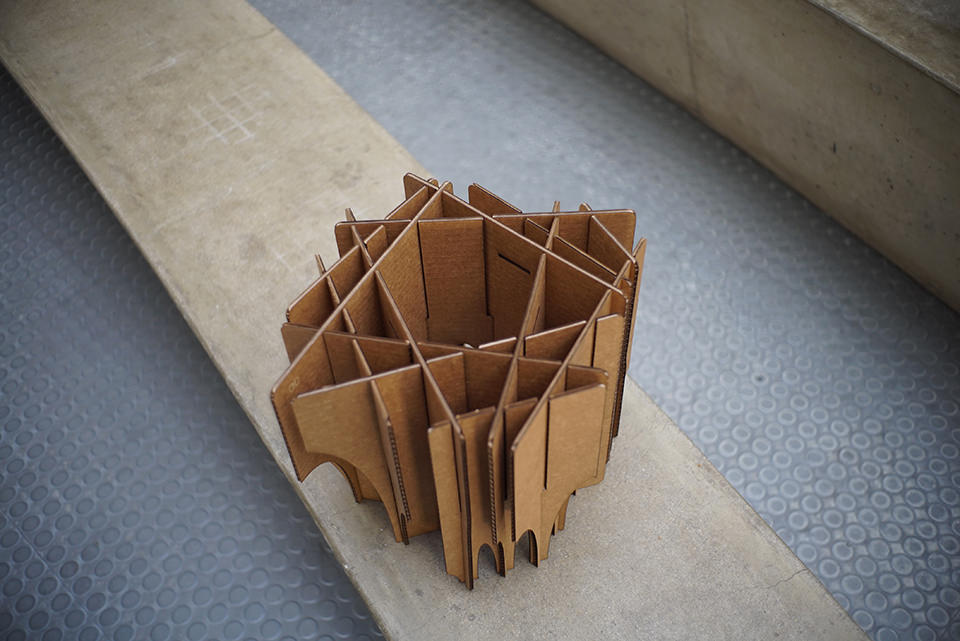Fig5. View from above. Random crossing interlocking.

I finished and put the object on my bedroom light stand. but...

• it was too big (bad propotion)
• the object was blocking too much light

So I did another iteration to overcome the errors. The final version has holes (which avoids to interfere with joints) to let light out.Fig8. Detail lighting of second trial.

## Appendix.

Rhino-python script for process automation.

```    ```import rhinoscriptsyntax as rs
import math

BOARD_THICKNESS = 0.1655 * 25.4 #mm
CUT_THICKNESS = cut_thickness * 25.4 #mm

def angle_cut_length(_degree):
'''
simple math to get the pitch of angled cut
'''

if _degree > 90 :
degree = 180 - _degree
else:
degree = _degree

inter_points = []
inter_indices = []
cut_lines = []

for i in range(len(lines)):
for j in range(i+1,len(lines)):
intr = rs.CurveCurveIntersection(lines[i],lines[j])
if isinstance(intr,(list,tuple)):
inter_points.append(intr)
inter_indices.append([i,j])

start_pos =[]
string_num = []
sx = 0
for i in range(len(lines)):
#for i in :

start_pos.append(sx+20)
if i<10 :
string_num.append('0'+str(i))
else:
string_num.append(str(i))

print 'No.'+str(i)
temp_indices =[]
temp_param = []
temp_intr_index = []

c = 0
for ii in inter_indices:

if ii==i:
temp_indices.append(ii)
temp_intr_index.append(c)

if ii==i:
temp_indices.append(ii)
temp_intr_index.append(c)

c += 1

crv_length = rs.CurveLength(lines[i])

#rs.Angle2() gets the angle from two lines in degrees.
intr_angles = [rs.Angle2(lines[i],lines[id]) for id in temp_indices]

crv_int_points =
[rs.Distance(rs.CurveStartPoint(lines[i]),inter_points[id])
for id in temp_intr_index]

db_points = [inter_points[id] for id in temp_intr_index]

print crv_int_points

#top,bottom

for j in range(len(crv_int_points)):
acl = angle_cut_length(intr_angles[j])/2.0

[sx+crv_int_points[j]-acl,height/2,0],
[sx+crv_int_points[j]+acl,height/2,0]
))

print str(i)+' connected_with ' + str(temp_indices[j])

if i < temp_indices[j] :
start_y = 0
else:
start_y = height/2.0